## 9 Aug 2012

### Tangent Lines and Slope Predictors in Edwards & Penney's Calculus

presentation of Edwards & Penney's work, by Corry Shores
[Search Blog Here. Index-tags are found on the bottom of the left column.]

[Central Entry Directory]
[Mathematics, Calculus, Geometry, Entry Directory]
[Calculus Entry Directory]
[Edwards & Penney, Entry Directory]

Edwards & Penney's Calculus is an incredibly-impressive, comprehensive, and understandable book. I highly recommend it.

[Search Blog Here. Index-tags are found on the bottom of the left column.]

[I your author am not a mathematician; I am merely an admirer of Edwards & Penney's wonderful calculus book. Please consult the text or other references to be certain about anything in the summary below. I mean this emphatically.]

Summary of
Edwards & Penney
Calculus

Chapter 2. Prelude to Calculus
Section 2.1. Tangent Lines and Slope Predictors

Recall the tangent problem. Consider circle O with point P on its circumference, making radius OP.[Thanks Edwards & Penney]

The line tangent to point P is "the straight line through P that is perpendicular to the radius (OP)."

When we have any general graph expressing y = f(x), we do not necessarily have some radius that will allow us to easily find a tangent. However, "the line tangent to the graph at the point P should be the straight line through P that has -- in some sense -- the same direction at P as the curve itself." (p.54a)

A line's slope tells us its direction. So to determine the line tangent to a curve, we will find a "slope-prediction formula" that will tell us the tangent's slope.

Example
"Determine the slope of the line L tangent to the parabola y = x2 at the point P(a, a2)." (p.54b)

Below we see the graph for y = x2[Thanks Edwards & Penney]

We can judge that the curve seems to be wanting to go in the direction of L at point P. We need now determine the slope of L.

Because we only know one point of line L [point P(a, a2)], we cannot calculate the slope. Instead we will begin with another line whose slope we are able to determine. The graph below displays secant line K. It passes through point P and as well through a nearby point Q(b, b2) along parabola y = x2.Notice here we have point P. It is at x-coordinate a. Point Q however is further down the x axis. It is at x-coordinate b. So between points a and b on the x axis, there is a change or increment of x, or delta-x, marked, Δx. We will call it h:

h = Δx = b - a

So recall the coordinates of Q.

Q(b, b2)

The first b is on the x-axis. Because it is found by adding to a the change in x, it is defined as

b = a + h

The b2 is found on the y axis. It is found through the function y = x2. So we define it as

b2 = (a + h)2

because (a + h) is how we get the x value for the function y = x2 at point Q.

And because the y coordinates are found through the function y = x2, the change in y between P and Q is

Δy = b2 - a2 = (a + h)2 - a2

The slope of a line we call m. And the slope is the change in y over the change in x.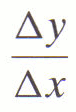We know both the change in y and the change in x for secant line K, so we can write the formulation.In the numerator, we multiply (a + h) by itself to get (a2 + 2ah + h2). We then subtract out the a's in the denominator to leave just h.We then subtract out the two a2's in the numerator to get 2ah + h2.Then finally we factor out the h from the numerator to get h(2a + h).We then cancel the h's from the numerator and the denominator, and thus the slope of secant K is

mPQ = 2a + h

Now consider if we move point Q toward point P along the curve, which by the way is the same as h (change in x) approaching zero.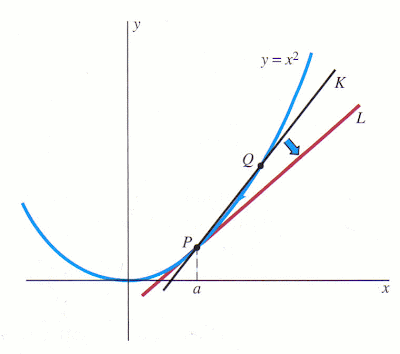Line K will continue to pas through points P and Q, pivoting around point P. As h approaches zero, secant line K moves closer to overlapping tangent line L. See this motion in the animation below.

We want to define tangent line L as "the limiting position of the secant line K." (p.55d) Then:
"As h approaches zero,
Q approaches P, and so
K approaches L; meanwhile,
the slope of K approaches the slope of L"
What we want to know is, as h approaches zero, what value is slope mPQ = 2a + h approaching? So we are looking for the "limiting value" of 2a + h.Here lim stands for "limit", and "h → 0" stands for "h approaches zero". What the above formulation asks is "What is the limit of 2a + h as h approaches zero?" (p.55b)

We first consider what if a were either 1 or -2.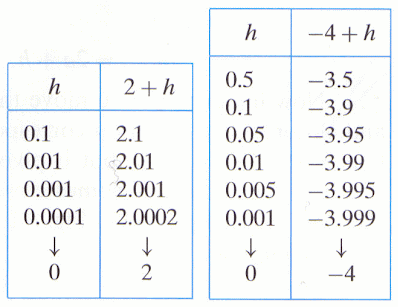We would see that if a were 1, then 2 + h tends toward 2 as h tends toward zero. And if a were -2, then 2 + h tends toward 2-4 as h tends toward zero.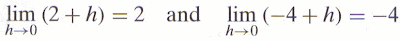So as we see for the values for slope 2a + h,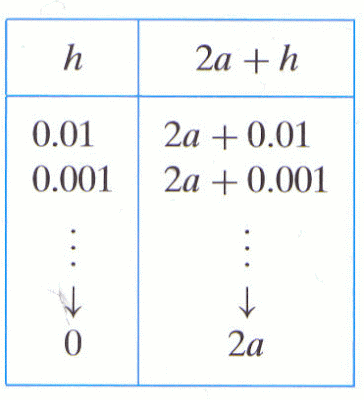we may say, more generally, thatThus the "slope m = m(a) of the line tangent to the parabola y = x2 at the point (a, a2) is given by

m = 2a

." (p.56d)

The above formula is the "slope predictor" for tangents to parabola y = x2. "Once we know the slope ofthe line tangent to the curve at a given point of the curve, we can then use the point-slope formula to write an equation of this tangent line." (p.56d)

Text summary and images from:
Edwards & Penney: Calculus. New Jersey: Prentice Hall, 2002, pp. 54-56.

#### 1 comment:

1.Thanks you very much ! This article help me very well .